1594604092

# Python機器學習：機器學習簡介

## 誰是此系列文章的目標讀者？

1. 已經能嫻熟使用 Python 基本程式設計的使用者
2. 想學習 Python 機器學習模型的使用者

## 誰可能不是此系列文章的目標讀者？

1. 從未接觸過 Python 的初學者
2. 已經嫻熟機器學習應用&深度學習領域實作的高階使用者

## 所以到底什麼是機器學習(Machine Learning)？

Machine learning is the science of programming computers so they can learn from data.

## 機器學習和人工智慧(AI)&深度學習(Deep Learning)的關係又是什麼呢？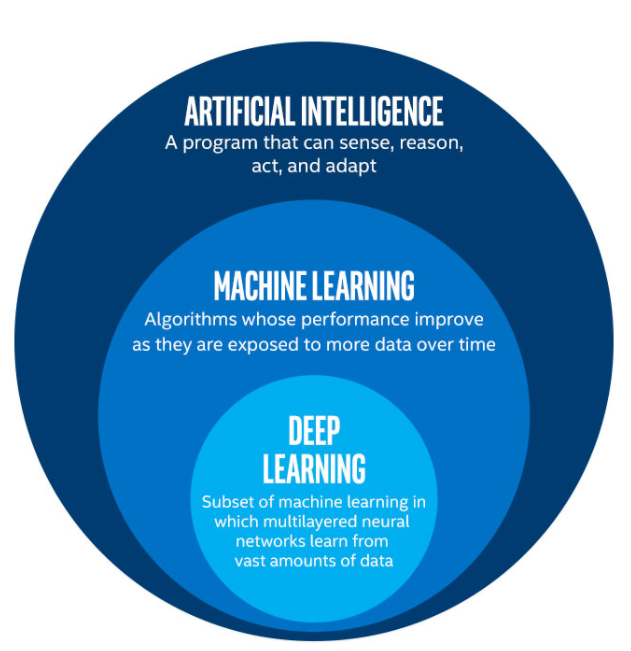#python #machine-learning

## Buddha Community1594604092

## 誰是此系列文章的目標讀者？

1. 已經能嫻熟使用 Python 基本程式設計的使用者
2. 想學習 Python 機器學習模型的使用者

## 誰可能不是此系列文章的目標讀者？

1. 從未接觸過 Python 的初學者
2. 已經嫻熟機器學習應用&深度學習領域實作的高階使用者

## 所以到底什麼是機器學習(Machine Learning)？

Machine learning is the science of programming computers so they can learn from data.

## 機器學習和人工智慧(AI)&深度學習(Deep Learning)的關係又是什麼呢？#python #machine-learning1594637220

## Python機器學習：如何處理加入類別變數的回歸模型

``````import pandas as pd

nba_data.info()
``````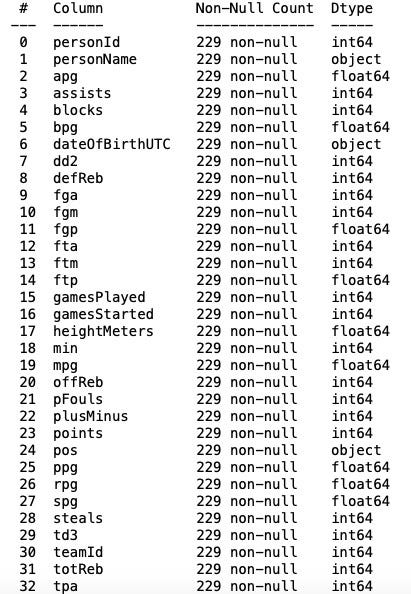## 籃球員的位置(Position)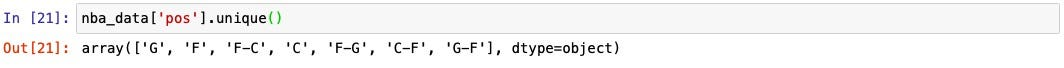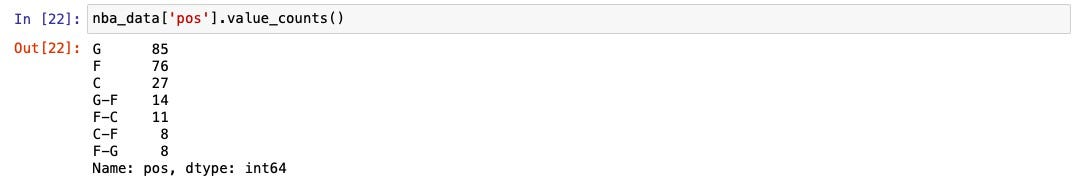``````pos_recategorized = {
'G': 'G',
'F': 'F',
'C': 'C',
'G-F': 'G',
'F-C': 'F',
'C-F': 'C',
'F-G': 'F'
}
nba_data['pos_recategorized'] = nba_data['pos'].map(pos_recategorized)
print(nba_data['pos_recategorized'].unique())
print(nba_data['pos_recategorized'].value_counts())
``````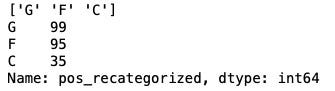``````import matplotlib.pyplot as plt
import seaborn as sns

sns.scatterplot(x = 'ppg', y = 'salary', hue = 'pos_recategorized', data = nba_data)
plt.show()
``````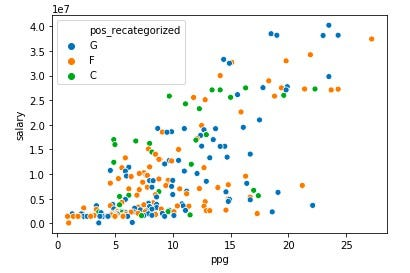#python #機器學習 #machine-learning1594673220

## Python機器學習：機器學習模型的計算複雜性

**計算複雜性（Computational complexity）**是電腦科學研究解決問題所需的資源，諸如時間（要通過多少步演算才能解決問題）和空間（在解決問題時需要多少記憶體），在演算法中常見到的大 O 符號就是表示演算所需時間的表達式。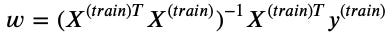## 因此只有在n變大的時候才有計算複雜性的議題！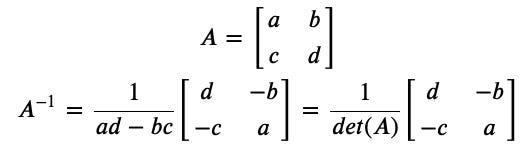``````import copy

A = [
[4, 7],
[2, 6]
]
det_A = A * A- A * A
neg_A = copy.deepcopy(A)
for i in range(len(neg_A)):
for j in range(len(neg_A)):
neg_A[i][j] = -A[i][j]
neg_A = A
neg_A = A
inv_A = [
[0, 0],
[0, 0]
]
for i in range(len(neg_A)):
for j in range(len(neg_A)):
inv_A[i][j] = neg_A[i][j] / det_A

print(inv_A)
``````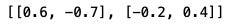``````import numpy as np

np.linalg.inv(A)
``````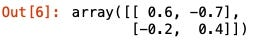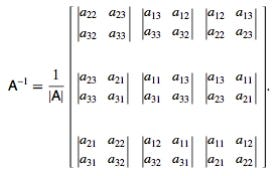#python #machine-learning #機器學習1594684020

## 使用 Scikit-Learn 的 Linear Regression 預測器找出 𝑤:

``````import pandas as pd
import numpy as np
from sklearn.model_selection import train_test_split
from sklearn.linear_model import LinearRegression

csv_url = "https://kaggle-getting-started.s3-ap-northeast-1.amazonaws.com/house-prices/train.csv"
X = housing["GrLivArea"].values.reshape(-1, 1)
y = housing["SalePrice"].values
X_train, X_validation, y_train, y_validation = train_test_split(X, y, test_size=0.33, random_state=42)
lr = LinearRegression()
lr.fit(X_train, y_train)
w = lr.coef_.copy()
w = np.insert(w, 0, lr.intercept_).reshape(-1, 1)
print(w)
``````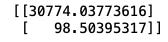## 使用 Gradient Descent 找出 𝑤：

``````def get_mse(X, y, w):
m = y.size
y_hat = X.dot(w)
y_reshaped = y.reshape(-1, 1)
err = y_hat - y_reshaped
se = err**2
sse = se.sum()
mse = sse / m
return mse

m = y.size
y_hat = X.dot(w)
y_reshaped = y.reshape(-1, 1)
err = y_hat - y_reshaped
``````
``````def gradient_descent(X, y, epochs, learning_rate):
n = X.shape
w = np.random.rand(n, 1)
j_history = []
w_0_history, w_1_history = [w[0, 0]], [w[1, 0]]
n_prints = 20
interval = epochs // n_prints
for i in range(epochs):
mse = get_mse(X, y, w)
j_history.append(mse)
if i % interval == 0:
w_0_history.append(w[0, 0])
w_1_history.append(w[1, 0])
print("{} iterations: w_0 = {}; w_1 = {}".format(i, w[0, 0], w[1, 0]))
return w, w_0_history, w_1_history, j_history
``````
``````from sklearn.preprocessing import PolynomialFeatures

poly = PolynomialFeatures(1)
X_poly = poly.fit_transform(X)
X_train, X_validation, y_train, y_validation = train_test_split(X_poly, y, test_size=0.33, random_state=42)
n_epochs = 300000
learning_rate = 1e-7
w, w_0_history, w_1_history, j_history = gradient_descent(X_train, y_train, n_epochs, learning_rate)
``````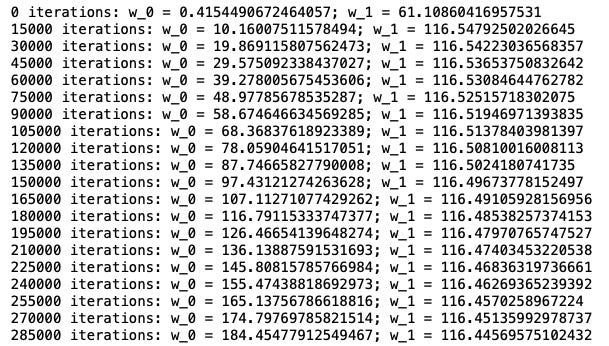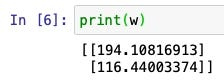``````import matplotlib.pyplot as plt

def get_gd_plots(j_history, w_0_history, w_1_history, best_w_0, best_w_1):
fig, axes = plt.subplots(1, 2, figsize=(12, 3))
axes.plot(range(len(j_history)), j_history)
axes.set_xlabel("Number of iterations")
axes.set_ylabel("MSE")
axes.set_title("MSE trend")
axes.scatter(w_0_history, w_1_history, marker="+")
axes.scatter(best_w_0, best_w_1, marker="x", s=200, color="red")
axes.scatter(w_0_history[-1], w_1_history[-1], marker="x", s=200, color="green")
axes.set_xlabel("\$w_0\$")
axes.set_ylabel("\$w_1\$")
axes.set_title("How does \$w_0\$ and \$w_1\$ converge")
#axes.set_ylim(0, 15)
plt.show()

get_gd_plots(j_history, w_0_history, w_1_history, 30774, 98.5)
``````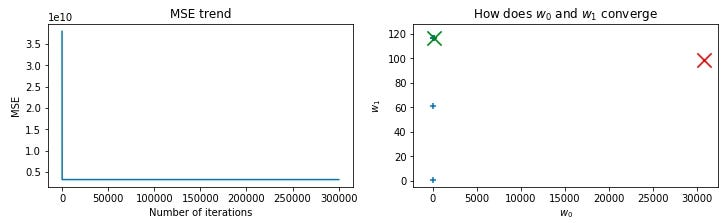#python #machine-learning #機器學習1594648020

## Python機器學習：機器學習中誤差的來源與正規化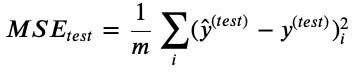h的誤差可能源於 Training error 與 Test error：

Training error 常被稱為 error或 bias

Test error 亦可被稱為 Generalization error, 被簡稱為 variance

``````import pandas as pd
from sklearn.model_selection import train_test_split
from sklearn.preprocessing import PolynomialFeatures
from sklearn.linear_model import LinearRegression
from sklearn.metrics import mean_squared_error

X = nba_data['ppg'].values.reshape(-1, 1)

degrees = range(1, 11)
training_errors = []
test_errors = []
for deg in degrees:
poly = PolynomialFeatures(degree = deg)
X_poly = poly.fit_transform(X)
y = nba_data['salary'].values
X_train, X_validation, y_train, y_validation = train_test_split(X_poly, y, test_size = 0.33, random_state = 42)
lr = LinearRegression()
lr.fit(X_train, y_train)
y_pred = lr.predict(X_train)
training_err = mean_squared_error(y_train, y_pred)
training_errors.append(training_err)
y_pred = lr.predict(X_validation)
test_err = mean_squared_error(y_validation, y_pred)
test_errors.append(test_err)
``````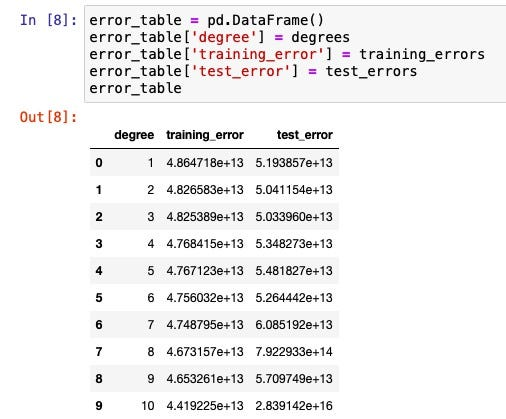#python #機器學習 #machine-learning